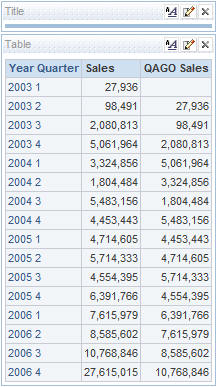# New to QlikView

Discussion board where members can get started with QlikView.

Announcements

#### Breathe easy -- you now have more time to plan your next steps with Qlik! QlikView 11.2 Extended Support is now valid through December 31, 2020. Click here for more information.Not applicable

## Is there any similar function in QlikView for Oracle BI function AGO

Hi ALL,

I have to convert the following expression in Oracle BI into QlikView:

---> sum(fo.sum_1) / nullif( Ago(sum(fo.sum_1), [Level Year], 1) , 0) * 100

[

AGO,MAGO,YAGO,QAGO

AGO(<measure>, <level>, <shift needed>)

]

[In Oracle, the SUM returns the sum of values of expr.]
[In Oracle, the NULLIF function compares expr1 and expr2.
If expr1 and expr2 are equal, the NULLIF function returns NULL. Otherwise, it returns expr1.]As you see above, QAGO has shifted the values by 1 quarter,

and AGO has shifted the values by 1 year -  Ago(sum(fo.sum_1), [Level Year], 1)

I am thinking I might have to do something in the script or am I wrong?

with t_ago as

(

select sum(fo.sum_1) as ago_sum_1,C.col1

from t_dim c

join t_fact fo on FO.col_key = C.col_key

join t_dim_date d on D.DATEKEY=FO.DATEKEY

where D.CYEARNAME = '2005'

group by C.col1

order by C.col1

)

SELECT col1

, col2

, col3

, col4

, ROUND(col / nullif( a.ago_sum_1 , 0) * 100,1) || '%' as "col5, %"

FROM

(

select C.col1

, sum(FO.sum_1) as col2

, sum(FO.sum_2) as col3

, sum(fo.sum_3) as col4

, sum(fo.sum_1) as col

from t_dim c

join t_fact fo on FO.col_key = C.col_key

join t_dim_date d on D.DATEKEY=FO.DATEKEY

where D.CYEARNAME = '2006'

group by C.col1

order by C.col1

) inview

JOIN t_ago a on a.col1 = inview.col1

2 RepliesMVP & Luminary

## Re: Is there any similar function in QlikView for Oracle BI function AGO

There are at least two ways. You can use the above() function in charts or the previous function in the load script. I've used both in the attached example so you can see how they work.

talk is cheap, supply exceeds demandNot applicable

## Re: Is there any similar function in QlikView for Oracle BI function AGO

Thank you so much, Gysbert Wassenaar!!!